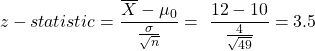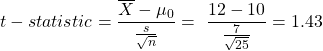Concept 15: Hypothesis Tests Concerning a Single Mean | IFT World
101 Concepts for the Level I Exam

# Concept 15: Hypothesis Tests Concerning a Single MeanWe use the following table to decide which test statistic and which corresponding probability distribution to use for hypothesis testing.

 Sampling from Small sample size Large sample size Normal distribution Variance known z z Variance unknown t t (or z) Non–normal distribution Variance known NA z Variance unknown NA t (or z)

You believe that the average returns of all stocks in the S&P 500 is greater than 10%. You draw a sample of 49 stocks. The average return of these 49 stocks is 12%. The standard deviation of returns of all stocks in the S&P 500 is 4%. Using a 5% level of significance, determine if your belief is correct.

Solution:

Step 1: State the hypothesis

H0: µ ≤ 10%

Ha: µ > 10%

Step 2: Calculate the test statistic

The population variance is known hence we will use z-statistic.Step 3: Calculate the critical value

This is a one-tailed test and we will be looking at the right tail. Using the Z –table and 5% level of significance

Critical value = Z0.05 = 1.65

Step 4: Decision

Since the test statistic (3.5) > critical value (1.65), we reject H0. Hence at 5% level of significance, your belief that the average returns of all stocks in the S&P 500 is greater than 10% is correct.

You believe that the average returns of all stocks in the S&P 500 is greater than 10%. You draw a sample of 25 stocks. The average return of these 25 stocks is 12% and the standard deviation of their returns is 7%. Using a 5% level of significance, determine if your belief is correct.

Solution:

Step 1: State the hypothesis

H0: µ ≤ 10%

Ha: µ > 10%

Step 2: Calculate the test statistic

The population variance is not known hence we will use t-statistic.Step 3: Calculate the critical value

This is a one-tailed test and we will be looking at the right tail. Using the t –table and 5% level of significance and degrees of freedom = 25 -1 = 24

Critical value = t24,0.05= 1.71

Step 4: Decision

Since the test statistic (1.43) < critical value (1.71), we cannot reject H0. Hence at 5% level of significance, your belief that the average returns of all stocks in the S&P 500 is greater than 10% is incorrect.

LIVE WEEKEND CLASSES *For 2024 Exam candidates*
This is default text for notification bar